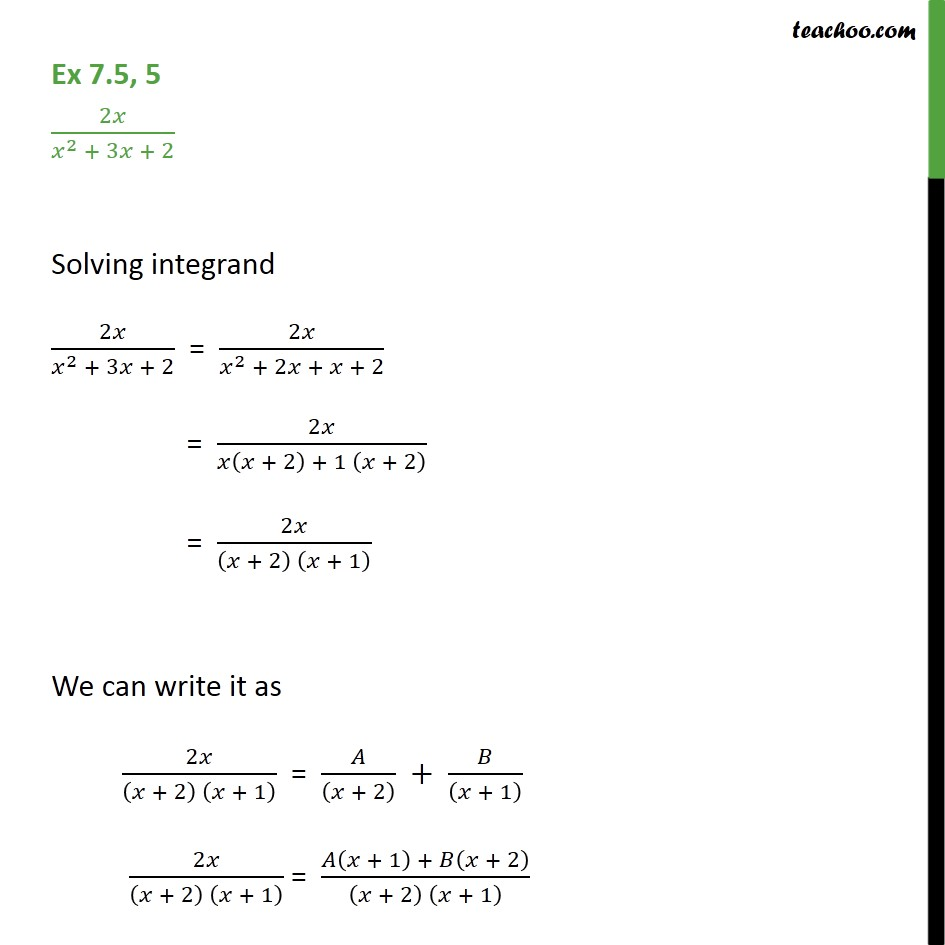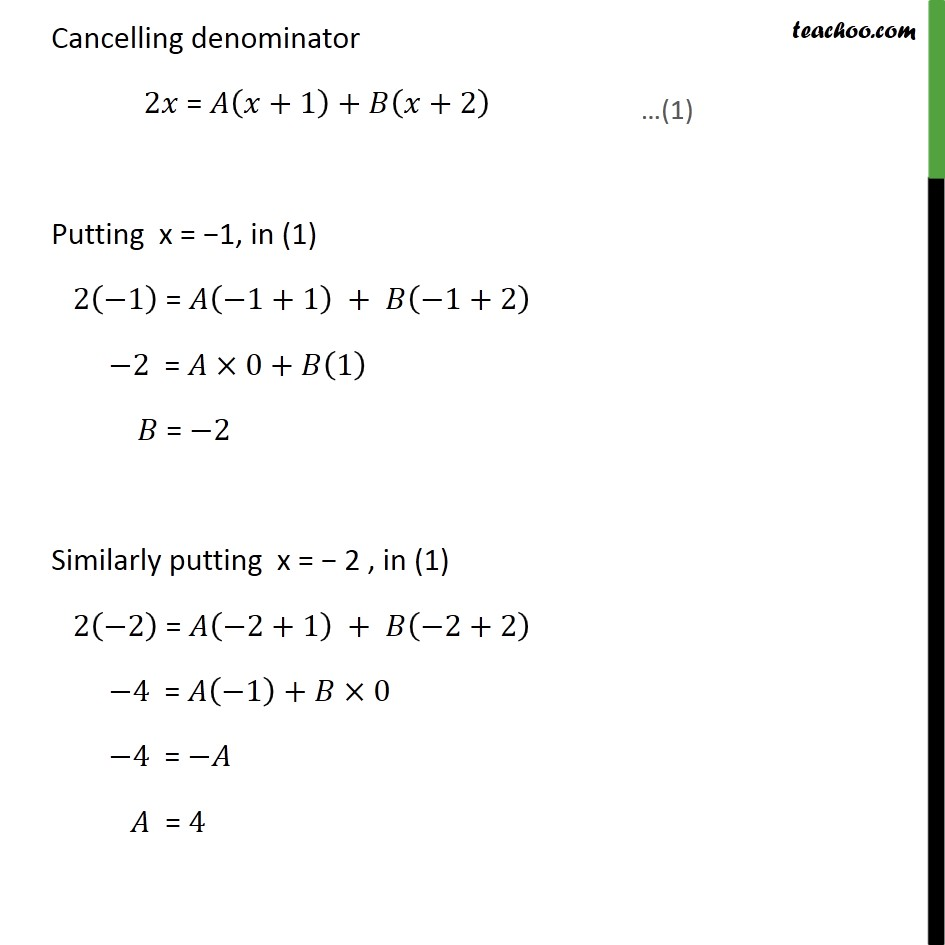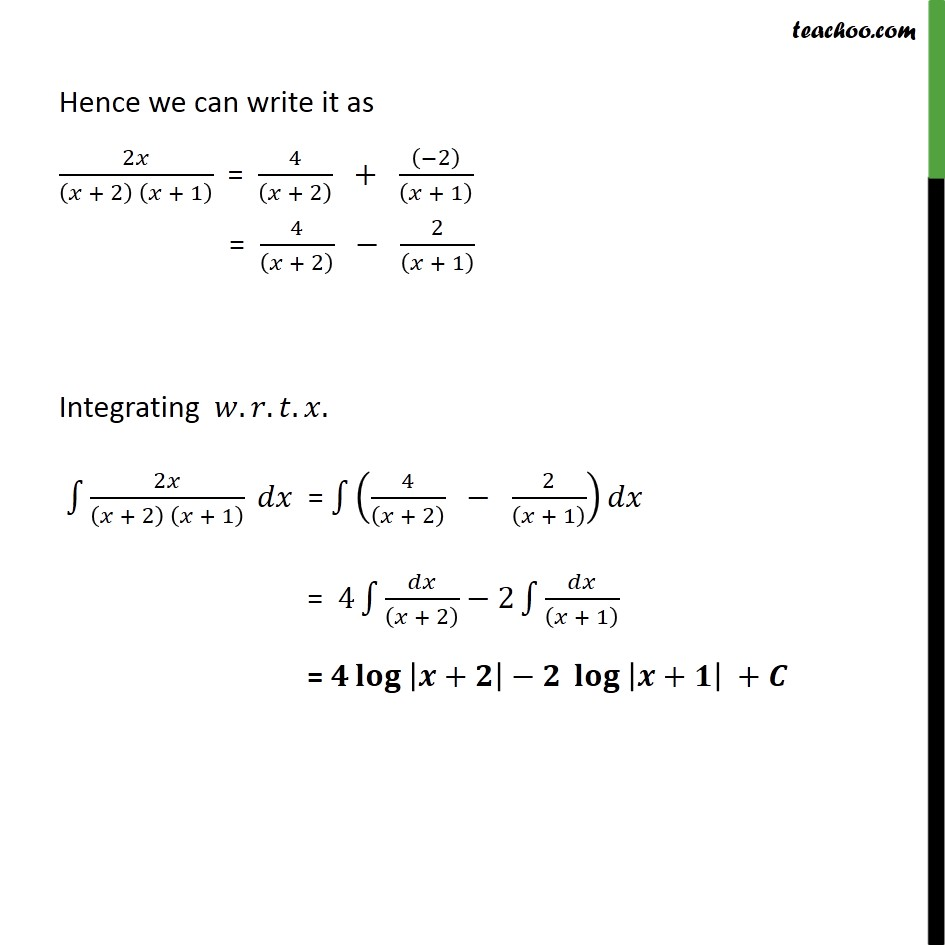Ex 7.5

Chapter 7 Class 12 Integrals
Serial order wiseLearn in your speed, with individual attention - Teachoo Maths 1-on-1 Class

### Transcript

Ex 7.5, 5 2𝑥﷮ 𝑥﷮2﷯ + 3𝑥 + 2﷯ Solving integrand 2𝑥﷮ 𝑥﷮2﷯ + 3𝑥 + 2﷯ = 2𝑥﷮ 𝑥﷮2﷯ + 2𝑥 + 𝑥 + 2﷯ = 2𝑥﷮𝑥 𝑥 + 2﷯ + 1 𝑥 + 2﷯﷯ = 2𝑥﷮ 𝑥 + 2﷯ 𝑥 + 1﷯﷯ We can write it as 2𝑥﷮ 𝑥 + 2﷯ 𝑥 + 1﷯﷯ = 𝐴﷮ 𝑥 + 2﷯﷯ + 𝐵﷮ 𝑥 + 1﷯﷯ 2𝑥﷮ 𝑥 + 2﷯ 𝑥 + 1﷯﷯ = 𝐴 𝑥 + 1﷯ + 𝐵 𝑥 + 2﷯﷮ 𝑥 + 2﷯ 𝑥 + 1﷯﷯ Cancelling denominator 2𝑥 = 𝐴 𝑥+1﷯+𝐵 𝑥+2﷯ Putting x = −1, in (1) 2 −1﷯ = 𝐴 −1+1﷯ + 𝐵 −1+2﷯ −2 = 𝐴×0+𝐵 1﷯ 𝐵 = −2 Similarly putting x = − 2 , in (1) 2 −2﷯ = 𝐴 −2+1﷯ + 𝐵 −2+2﷯ −4 = 𝐴 −1﷯+𝐵×0 −4 = −𝐴 𝐴 = 4 Hence we can write it as 2𝑥﷮ 𝑥 + 2﷯ 𝑥 + 1﷯﷯ = 4﷮ 𝑥 + 2﷯﷯ + −2﷯﷮ 𝑥 + 1﷯﷯ = 4﷮ 𝑥 + 2﷯﷯ − 2﷮ 𝑥 + 1﷯﷯ Integrating 𝑤.𝑟.𝑡.𝑥. ﷮﷮ 2𝑥﷮ 𝑥 + 2﷯ 𝑥 + 1﷯﷯﷯ 𝑑𝑥 = ﷮﷮ 4﷮ 𝑥 + 2﷯﷯ − 2﷮ 𝑥 + 1﷯﷯﷯﷯𝑑𝑥 = 4 ﷮﷮ 𝑑𝑥﷮ 𝑥 + 2﷯﷯﷯−2 ﷮﷮ 𝑑𝑥﷮ 𝑥 + 1﷯﷯ ﷯ = 𝟒 𝐥𝐨𝐠 ﷮ 𝒙+𝟐﷯﷯−𝟐 𝐥𝐨𝐠 ﷮ 𝒙+𝟏﷯﷯ +𝑪